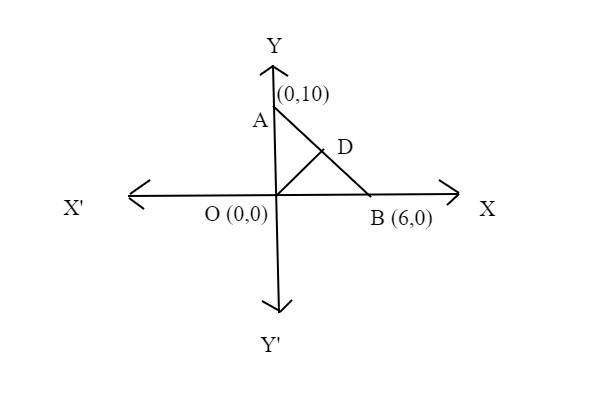Courses
Courses for Kids
Free study material
Free LIVE classes
MoreLIVE
Join Vedantu’s FREE Mastercalss

# Write the coordinates of the orthocentre of the triangle whose vertices are at (0,0),(6,0) and (0,10).Verified
362.4k+ views
Hint: Let us make use of the definition of orthocentre which says that it is nothing but the point of intersection of the altitudes.

To solve this, let us consider a right angled triangle with the given vertices (0,0),(6,0),(0,10).
So, on constructing a right angled triangle, it would beNow, we know from the definition that orthocentre is nothing but the point of intersection of all the altitudes of the triangle,
Now since it is a right angled triangle, we can write that the sides AO, OB are nothing but the altitudes of the sides OA and OB and OD is the altitude of the side AB.
So, from this, we can clearly observe that all these three altitudes AO,OD,OB intersect at the point O(0,0), this is nothing but the origin.
So, from this we can write that the orthocentre of the triangles with vertices (0,0),(6,0),(0,10) is (0,0).
Note: In this question we have not been given that the triangle is a particular type of triangle, so we have considered it as a right angled triangle, in case if it was given that the triangle is an isosceles or Equilateral triangle, then we will solve the problem accordingly.
Last updated date: 30th Sep 2023
Total views: 362.4k
Views today: 5.62k